SELF 30 S.B. Karavashkin and O.N. Karavashkina“The same as in case of speed, in the theory of relativity instead the three-dimensional vector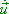we introduce … the four-dimensional vector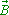with the components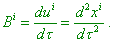(14)
 In the special theory of relativity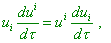(15)
 so from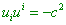(16)
 through differentiation we yield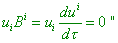(17)
 [6, p. 113], wheremeans the intrinsic time of accelerated body. To understand that the definition (14) is artificial, follow its derivation which, as we reveal, is grounded on the very general properties of warped space, although in SR, with its Euclidean spatial metric, this obviously is excessive and only corroborates that relativists know the discrepancy in this aspect of their theory. None the less, it is easy to check that relativists introduce the acceleration in frames of invariant differential operations as follows: “Let at each point of the curve xk = xk (t)  there is given a vector with the components ai  (it still is not the vector of acceleration - Authors). Choose arbitrarily a permanent point on the curve P and graph the second assemblage of vectors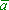i (P') , where P'  is any point on the curve, by way of parallel transfer of the vector ai (P) along the curve. At the point P,i   and  ai  coincide: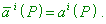(18)
 Then with the help of relation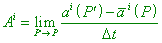(19)
 we can invariantly determine the new vector, as in the numerator we see the difference of two vectors at one point. From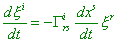(20)
 (page 63 of the cited work - Authors) and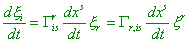(21)
 (page 65 of the cited work - Authors) it follows that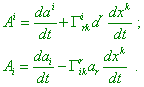(22)
 If we substitute instead t the length of arc s and instead ai  - the tangential vector ui = dxi / ds , we will yield in this way the vector of ‘acceleration’ whose components Bi  coincide with the left part (of the equation of geodesic line - Authors):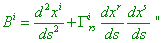(23)
 [6, p. 89- 90]. We can see from this derivation that (14), just as (23), does not account the above features differentiation within Minkowski 4-D metric caused by the features of Lorentz transformation. All derivatives, second-order in that number, are determined, proceeding from the mind that the operation is true, though it has to be strongly proven. And we see in the derivation the ungrounded transitions from the time coordinate to that spatial changing not only the meaning of transformations but also the dimension of the result. But with it, just the prototype of (23) Pauli took as the basis when finding the concept of acceleration, and not in vain he selected this word by quotation marks. The vector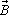has no relation to the real acceleration in IRFs, as well as its relationship with the 3 D vector is quite strange [6, p. 113]: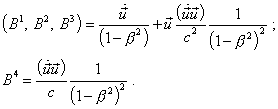(24)
 It follows from (24) that in case of considered problem of direct accelerated motion of the body with respect to the stationary RF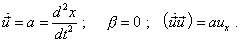(25)
 Thus,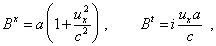(26)
 which means that in relativistic conception, the acceleration time-constant as to IRFs is basically impossible. Moreover, as with the accelerated motion  ux  grows with time, Bx  also grows with time without additional affection, except of force which has to provide the constant acceleration by the statement of problem. This definition of acceleration is not some particular representation of Pauli. Levich defines, similarly, “the four-dimensional acceleration wa as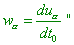(27)
 [7, p. 219], where t0   is the intrinsic time. Thereupon he comes to the same expressions (24) that define the 4 D ‘acceleration’.

Contents: / 29 / 30 / 31 / 32 / 33 / 34 / 35 / 36 / 37 / 38 / 39 /